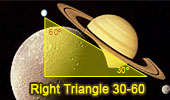Special Right Triangle 30 60 Theorems and Problems - Table of ContentEuclid's Elements Book I, 23 Definitions. One-page visual illustration. Euclid's Elements Book. Index Geometry Problem 1517 and a Thematic Poem. Unlocking Triangle Side Length: Solving with a Median and Two Angles. Difficulty Level: High School. Geometry Problem 1514 and a Thematic Poem. Discover the Secret to Finding Distances in Regular Hexagons with Interior Squares. Difficulty Level: High School. Geometry Problem 1461. Quadrilateral, Triangle, Angles, 30-60 Degree, Congruence, Auxiliary Lines. Geometry Problem 963. Right Triangle, 30-60-90 Degrees, Angle Bisectors, Metric Relations. Geometry Problem 956. Two Equilateral Triangles, Center, Collinear Points, Midpoint, Right Triangle, 30, 60, 90 Degrees. Proposed Problem 405. Quadrilateral, 60, 75, and 135 degrees, Midpoint. Proposed Problem 304. Triangle, Angle bisector of 120 degrees. Proposed Problem 302. Triangle, Angle bisector of 60 degrees. Proposed Problem 102.Equilateral Triangle Area, Interior Point. Proposed Problem 44. Angles and triangles, 60 degrees angle. Proposed Problem 43. Angles and triangles. Proposed Problem 42. Angles and triangles, 60 degrees angle.